Atnaw, S. M., Sulaiman, S. A., Singh, L., Wahid, Z. A., and Ku Mohammad Faizal Bin Che Ku Yahya , C. (2017). "Modeling and parametric study for maximizing heating value of gasification syngas," BioRes. 12(2), 2548-2564.

#### Abstract

There are a number of experimental and theoretical studies on the energy conversion of oil palm derivative biomass. Moreover, the potential of this abundant biomass residue for renewable energy in major producing countries in Southeast Asia has been well documented. In this study, the results of an equilibrium model of downdraft gasification of oil palm fronds (OPF), developed using the Aspen Plus chemical process simulator software, and its validation are presented. In addition, an optimization of the major output parameter of importance (i.e., the higher heating value of syngas) with respect to the main operating parameters (i.e., temperature, equivalence ratio (ER), and moisture content) was performed. The response surface method (RSM) was used to determine the mathematical relationship between the response of interest, which was the heating value of syngas, and the operating conditions. This method was used to further determine the conditions that would lead to optimum higher heating values of syngas. Optimum values identified by RSM were: oxidation zone temperature of 1000 °C, moisture content in the range of 4%, and an equivalence ratio of 0.35. These optimum operating conditions and the corresponding higher heating value of syngas were found to correspond with the experimental results.

Modeling and Parametric Study for Maximizing Heating Value of Gasification Syngas

Samson Mekbib Atnaw,a,* Shaharin A. Sulaiman,b Lakhveer Singh,a

Zularisam A. Wahid,a and Che Ku Mohammad Faizal Bin Che Ku Yahya a

There are a number of experimental and theoretical studies on the energy conversion of oil palm derivative biomass. Moreover, the potential of this abundant biomass residue for renewable energy in major producing countries in Southeast Asia has been well documented. In this study, the results of an equilibrium model of downdraft gasification of oil palm fronds (OPF), developed using the Aspen Plus chemical process simulator software, and its validation are presented. In addition, an optimization of the major output parameter of importance (i.e., the higher heating value of syngas) with respect to the main operating parameters (i.e., temperature, equivalence ratio (ER), and moisture content) was performed. The response surface method (RSM) was used to determine the mathematical relationship between the response of interest, which was the heating value of syngas, and the operating conditions. This method was used to further determine the conditions that would lead to optimum higher heating values of syngas. Optimum values identified by RSM were: oxidation zone temperature of 1000 °C, moisture content in the range of 4%, and an equivalence ratio of 0.35. These optimum operating conditions and the corresponding higher heating value of syngas were found to correspond with the experimental results.

Keywords: Syngas; Higher heating value; Gasification; Biomass; Palm oil residues

Contact information: a: Universiti Malaysia Pahang, Faculty of Engineering Technology, Gambang, Pahang, 26300, Malaysia; b: Universiti Teknologi Petronas, Department of Mechanical Engineering, Tronoh, Perak 31750, Malaysia; *Corresponding author: mekbibsams@ump.edu.my

INTRODUCTION

Malaysia and Indonesia are the two major palm oil producing countries, covering more than 85% of the world’s total supply (Hansen et al. 2011; Umar et al. 2014). Hence, a tremendous amount of sustainable and renewable raw material exists in these two countries. Malaysia alone was forecasted to have higher than 40% of the material in 2015 (Sumathi et al. 2008). From 2005 to 2009, palm oil plantation coverage reached about 14% of the total land area in the country, with an average growth rate of 3.9% (Hansen et al. 2011). Such an ample and sustainable resource for renewable energy could be used for bringing renewable energy to the mainstream energy supply in Malaysia (Mohammed et al. 2010; Chang 2014).

The process of gasification is relatively complicated and involves a large number of chemical and physical processes (Baruah and Baruah 2014). The gasification process takes place in four stages called drying, pyrolysis, oxidation, and reduction. A number of studies had been carried out in the past on modelling of the thermochemical conversation of various biomass materials (Muilenburg et al. 2011; Bates et al. 2016). Phenomenological models that represent the various chemical processes in each stage of gasification using mathematical relations (Muilenburg et al. 2011; Sulaiman et al. 2012; Bates et al. 2016) are more commonly applied than the black box modelling approach that use Artificial Neural Network (ANN) (Puig-Arnavat et al. 2013; Rodrigues et al. 2016). These models can be placed into two main classes: equilibrium models that assume all major reactions of pyrolysis and gasification to reach chemical equilibrium during the gasification process, and kinetic models, which involve kinetic rate equations. In the current study the equilibrium modelling approach is used, as it has been reported by many authors to satisfactorily predict the gasification process. Most of the modelling work in the literature has focused on predicting the composition of resulting syngas from the gasification process depending on values of operating conditions. Yet most of the studies do not focus on optimizing the heating value of produced syngas and determining the optimum operating conditions.

Biomass residues from the oil palm industry include empty fruit bunches (EFB), palm shells, palm oil mill effluent (POME), trunks, and oil palm fronds (OPF). Previous studies on the downdraft gasification of OPF showed that it has a high potential as a feedstock for thermal gasification, with an output comparable to that of sugarcane and woody biomass materials. In a previous study, the authors presented the results of an experimental study on the influence of the reactor temperature, moisture content, and equivalence ratio on producer gas composition, temperature distribution along the gasifier bed, tar generation, higher heating value (HHV), and overall gasification efficiency (Atnaw et al. 2011; Sulaiman et al. 2012; Atnaw et al. 2013a). The results from the experimental study on the downdraft gasification of OPF was found to be comparable in terms of output with that of woody materials. In addition, the results of the equilibrium model of gasification were validated using experimental data, and the results of the optimization model that was used to determine the combination of operating conditions that could give the optimum (i.e., highest) output were presented. The current paper focuses on the optimization of a response of interest (i.e., higher heating value of syngas), which is dependent upon a number of operating conditions of the gasification process.

MODELING AND EXPERIMENTAL

Equipment and Materials

A downdraft gasifier (Multi Products Supply Enterprise sdn. Bhd., Penang, Malaysia) with a design capacity of 50 kW thermal output and a cylindrical reactor 1000 mm in height and 400 mm in diameter was used for the experimental study. In addition, a number of cooling and cleaning apparati were provided downstream of the gasifier before the syngas sampling point. To develop the optimum and safest method of operating the gasifier, and to put in place all necessary measuring instruments, a considerable amount of time and effort was spent during the initial development, testing, and commissioning. The details of the experimental setup and the operation procedure are available in the previous report (Atnaw et al. 2013b). The gasification experiments were conducted at average ambient air of 30 °C and at 80% relative humidity.

Modeling

An equilibrium model of downdraft gasification was developed using the Aspen Plus (version 8.0, Kuantan, Malaysia) process simulator software. A four-zone gasification model, which used four number unit operation models to model each zone of the gasification process (drying, pyrolysis, oxidation, and reduction), was developed. The flow sheet of the model that was developed shows a schematic of the reactors and the various streams of the four-zone gasification model and is presented in Fig. 1. The drying zone was modeled using the Rstoic reactor block of Aspen, while the pyrolysis zone of gasification was modeled using the RYield reactor. As the oxidation and reduction zones of the gasification process were known to occur at different temperature values, the two zones were modeled using two separate Gibbs reactors, at 850 and 650 °C, respectively. The conventional gaseous components considered in the modeling of the gasification process were: H2, O2, CO, CO2, H2O, N2, CH4, and S. From the different stream classes in Aspen, the conventional stream class was used for the gaseous reactants and product, whereas the char produced from gasification was defined as pure carbon. This assumption was adequately valid, as char has been known to be composed mainly of carbon, which was also confirmed experimentally from the ultimate analysis. Therefore, a conventional solid stream class was used for the char. The OPF was defined as a non-conventional solid using the component attribute features of Aspen.

The physical and chemical properties of OPF from the proximate and ultimate analyses were used in defining OPF as a non-conventional solid in Aspen. Shown in Table 1 is a summary of the physical and chemical properties of OPF. The initial estimations of the temperature of the various gasification zones and other input parameters are given in Table 2. The summary of the reactor blocks used to model each zone of gasification and the specific feature of each block are shown in Table 3. The optimization of the model results was performed using the response surface method (RSM).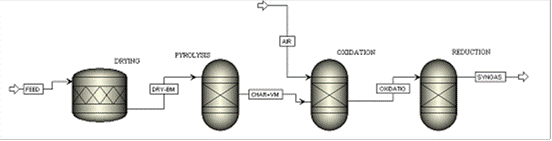Fig. 1. Schematic of the downdraft gasification model

Table 1. Physical and Chemical Properties of OPF*dry weight basis

Table 2. Model Input ParametersTable 3. Summary of Reactor Blocks Used in the ModelStatistical Analysis

The sum square deviation method of statistical analysis was used to estimate the accuracy of simulation predictions, and to analyze the level of agreement between experimental data and model results.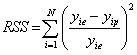(1)

In Eq. 1, is experimental composition value of each syngas component and stands for predicted composition from the simulation work. The average root sum square (MRSS) error was calculated considering the total number of data (N), and calculation of the mean error was determined as shown below: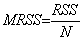(2)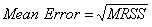(3)

RESULTS AND DISCUSSION

The typical simulation results corresponding to the operating conditions of an air fuel ratio of 1.71 kg air/kg OPF, an oxidation zone temperature of 850 oC, and initial fuel moisture content of 15% are given in Table 4. The lower heating value (LHV) of syngas was calculated based on the predicted average composition of the combustible gas components (CO, CH4, and H2), and was 4.86 MJ/Nm3. In addition, the experimental results from previous literature of the average syngas composition for the downdraft gasification of various biomass types are given in Table 5. These values and the simulation results were not directly comparable, as characteristics such as the type of feedstock, operating parameters, as well as the design and operation of the gasifiers differed. However, the typical syngas composition and heating values from the experimental data reported in previous studies were used as an initial reference for the model results. The sum of squared deviation method of statistical analysis was used to assess the accuracy of the simulation results against the other studies’ values. The validation of the simulation results with actual experimental data for the OPF downdraft gasification is presented later in this paper.

Table 4. Model Input Parameters and Average Simulation ResultsTable 6 shows results of the data analysis against the typical literature values, using the root-mean-square-deviation method.

Table 5. Typical Syngas Composition Values for Gasification of Various Types of Biomass*N/A – Not Available

From this accuracy calculation, it can be seen that the model predictions for the compositions of CO, CO2, H2, and CH4, as well as the heating value of syngas were in good agreement with previous results. The calculated mean error values between the predicted and the literature results are given in Table 4. As shown in Table 6, with the exception of CH4, the predicted syngas composition values and lower heating values were in good agreement with the results from previous studies.

Table 6. Mean Error in Fractions between Equilibrium Model Predictions and Typical Literature Values

 CO CO2 H2 CH4 N2 LHV 0.12 0.18 0.18 0.69 0.09 0.20

A sensitivity analysis on the influence of various operating conditions was performed using the equilibrium model of gasification developed with Aspen Plus. The average syngas compositions were predicted from the model, and the results of the effects of the oxidation zone temperature, equivalence ratio (ER), and moisture content are presented.

Influence of Reactor Temperature

One of the major parameters influencing the syngas composition obtained from gasifiers is the reactor temperature. The effect of the reactor temperature on the predicted gas composition was performed by varying the oxidation zone temperature for the range of 500 to 1200 °C using the Aspen sensitivity analysis tool. Figure 2 shows the variation in the volume fraction of the component gases in syngas with the oxidation zone temperature predicted by the model, with the operating conditions held constant at an equivalence ratio of 0.36 and fuel moisture content of 15%.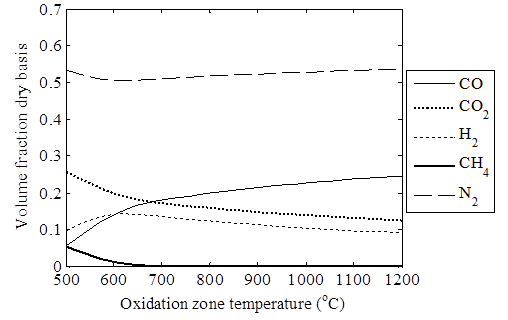Fig. 2. Oxidation zone temperature values versus predicted syngas composition in dry volume fraction

As shown in Fig. 2, the predicted H2 composition increased from about 10% to roughly 15% maximum for the oxidation zone temperature range of 500 °C to 700 °C. The H2 composition was found to be at a maximum at the oxidation zone temperature value of 600 °C, and steadily decreased to a value of about 10% for the temperature range of 500 to 1200 °C. Detournay et al. (2011) reported a similar steady decreasing trend in the composition of H2 in syngas at higher temperatures. They suggested that it had to do with a shifting of the reaction equilibrium towards CO and the H2O production at high temperatures, leading to a lower H2 composition.

The composition of CO in syngas was predicted to undergo a sharp increase from 500 °C up to approximately 700 °C, and then increase steadily until it reached 1200 °C, as shown in Fig. 2. The CO composition increased from about 5% to 25% for the temperature range of 500 to 1200 °C; CO was the only component that showed a significant response to the oxidation zone temperature above 800 °C. Considering that the CO composition made a remarkable contribution to the heating value of syngas, operation in the higher temperature range above 800 °C would be advantageous. The CO and CO2 compositions were predicted to have an inverse relation. The CO2 composition was predicted to steadily decrease with the reactor temperature, while the composition of CO increased. This increase in CO composition at higher reactor temperatures was mainly due to the fact that the endothermic reactions that produce CO are more favored at high temperatures. The inverse relation also showed that the product gases CO and CO2 were competing for the same reactant component, namely the carbon. Similar trends have been reported in the work of different authors (Zainal et al. 2001; Mathieu and Dubuisson 2002; Zainal et al. 2002). The composition of the inert component N2 was predicted to be relatively high at lower reactor temperatures, where the steady production of other gas components would be just starting, and showed a decreasing trend up to a temperature of 600 °C. For temperatures above 600 °C, the N2 composition remained nearly constant at an average value of 51%, as expected from its inert characteristics. Figure 2 also shows that the composition of CH4 dropped sharply from an initial value of about 6% to a trace amount in the oxidation zone temperature range of 500 to 700 °C. The predicted amount of CH4 was much lower in the higher temperature range, which could have been due to the promotion of endothermic reactions, such as steam reforming the consuming methane. Considering the relative composition of the syngas components, the optimum range of operation in terms of oxidation zone temperature was in the range of 850 to 1200 °C.

The results from the gasification experiments performed in the current study were used to validate the gas composition and heating value predictions of the product gas from the equilibrium model. The sum of squared deviations method of statistical analysis was used to calculate the accuracy of the simulation results compared to the experimental data. Generally, the CH4 concentrations predicted by the equilibrium model were very minimal (only trace amounts), while values obtained from the actual experiments were typically in the range of 0.8% to 2.8%. Similar results were reported in studies for the prediction of CH4 composition in syngas utilizing the equilibrium model of gasification (Zainal et al. 2001; Gautam 2010). Gautam (2010) reported that the equilibrium model predicted only trace amounts of CH4 for temperatures above 800 °C. This result in the prediction of CH4 composition was in close agreement with the results of the current study, as shown in Fig. 2. Similarly, the equilibrium model of downdraft gasification developed by Zainal et al. (2001) predicted only trace amounts of CH4 (0.64%) as compared to the experimental values in the range of 1.58% that were reported in the current study.

Shown in Table 7 is a comparison of the predicted and experimental results for the gas components CO, CO2, H2, and N2 in syngas. The mean error values obtained between the prediction and experimental value for the composition of each gas component and lower heating value is given in Table 8. The concentration of CO in both the experimental results and the results prediction from the equilibrium model increased with temperature, as shown in Table 7. The model predictions for the CO composition in syngas for the oxidation zone temperature above 850 °C were found to be lower than the experimental results. This could be attributed to the fixed reduction zone temperature of 782.78 °C used in the model, as the sensitivity analysis in Aspen was performed by varying only one parameter (i.e., oxidation zone temperature) while keeping other parameters (moisture content, air-fuel ratio, pyrolysis zone temperature, and reduction zone temperature) constant. However, the predicted results were obtained with reasonable accuracy, with a mean error of 0.19 as compared to experimental data for predicting the variation in the CO composition with temperature.

The predicted CO2 composition was found to be in close agreement with the experimental data, as shown in Table 7. The equilibrium model predicted the composition of CO2 in syngas with a mean error of 0.08, as shown in Table 8. The variations in the N2 and H2 composition between the predicted and experimental values were shown to be in good agreement with the mean errors of only 0.09 and 0.12, respectively, as compared to the experimental results.

Table 7. Comparison of Predicted and Experimental Gas Compositions at Different TemperaturesP* = Predicted values, E* = Experimental values

Figure 3 shows the comparison of the predicted and experimental values of the LHV of syngas over the range of oxidation zone temperatures between 700 and 1200 °C. As shown in Fig. 3, the heating value calculated from the gas composition predicted by the equilibrium model of gasification was in good agreement with the experimental data. Both the equilibrium model and the experimental results showed a slight increase in the LHV of syngas with temperature. A mean error of 0.13 was obtained between the predicted and experimental values of LHV for the variation in the oxidation zone temperature between 700 and 1200 °C. This showed that the equilibrium model of prediction was in good agreement with the experimental data at a satisfactory level of accuracy as compared to mean error values in other studies (Zainal et al. 2001).

Table 8. Mean Error Fractions between Predicted and Experimental Values

 Predicted Parameters CO H2 CO2 N2 Mean Error 0.19 0.12 0.08 0.09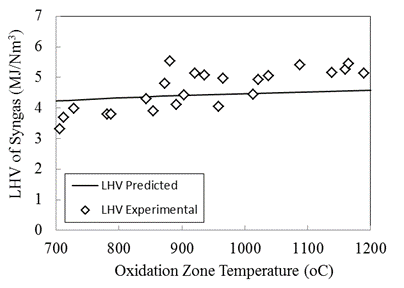Fig. 3. Comparison of predicted and experimental values of LHV of syngas with oxidation zone temperature

Influence of Equivalence Ratio

The influence of the equivalence ratio of gasification on the predicted syngas composition from the equilibrium model of downdraft gasification of OPF was determined by varying the equivalence ratio from 0.2 to 0.55. From the thermodynamic calculations, the theoretical equivalence ratio for the gasification of OPF was found to be 0.36. Hence, the predicted values of syngas composition in the range near this value were expected to be closer to the experimental values.

Shown in Fig. 4 is the variation in the predicted gas composition of syngas with its equivalence ratio. As shown, generally the percentages of CO and H2 were predicted to be higher at low equivalence ratios, as the modeling was performed at a set value of the oxidation zone temperature (850 °C) that could not be practically achieved at lower equivalence ratio values. Therefore, the predicted values for the equivalence ratio above the theoretical minimum value of 0.36 would be of practical interest.

As shown in Fig. 4, the compositions of CO, H2, and CH4 were predicted to decrease, while the compositions of CO2 and H2 increased with the increase in equivalence ratio. An inverse relationship between the concentrations of CO and CO2 was observed, with the inert component N2 showing a nearly liner increase with equivalence ratio, as could be expected.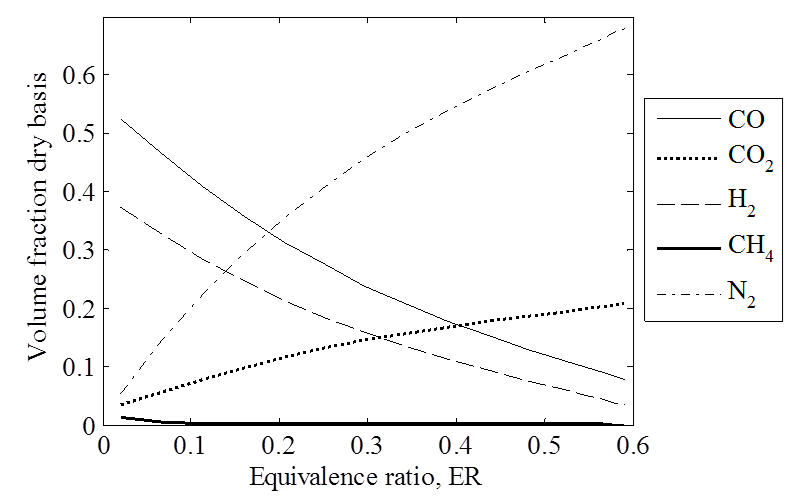Fig. 4. Equivalence ratio values versus predicted syngas composition from simulation in dry volume fraction (at Toxdn =850 °C, MC = 15%)

Shown in Table 9 is a comparison of the predicted and experimental variation of CO, CO2, H2, and N2 compositions in the product gas for the equivalence ratios between 0.35 and 0.59. The experimental data points obtained for the gasification experiments at the different values of equivalence ratio were used for comparison with the predicted results.

Table 9. Comparison of Predicted and Experimental Gas Compositions at Different Equivalence Ratios of GasificationP* = predicted values, E* = experimental values

The mean error values obtained between the predicted and experimental values for the composition of each gas component and for the lower heating values are given in Table 10. The equilibrium model predictions for the compositions of CO, CO2, and N2 were found to be in good agreement with the experimental results, as shown in Table 9. Table 9 also shows that the H2 concentration predicted by the model was found to be slightly higher than the experimental data for the equivalence ratio between 0.35 and 0.41. Such higher predictions of the H2 composition in syngas from the equilibrium model of gasification have been reported in other studies as well (Zainal et al. 2001; Gautam 2010). The reason for such higher prediction by the model could be due to the assumption that all the tar produced during gasification will be broken down to the simple hydrocarbon forms (namely H2 and CH4). In addition, the equilibrium model of gasification predicted the composition of CH4 in syngas to be in trace amounts, while experimental results in the range of 0.66% to 1.83% were obtained for the range of equivalence ratios considered.

Table 10. Mean Error Fraction between Predicted and Experimental ValuesShown in Fig. 5 is the comparison of the predicted and experimental values of the LHV of syngas for the range of equivalence ratios between 0.35 and 0.59. For the practically optimum range of equivalence ratios from 0.36 to 0.45, the heating value estimated based on predicted syngas composition decreased from 5.5 MJ/Nm3 to 3.6 MJ/Nm3. Figure 5 shows that the predicted and experimental results were in good agreement, and that the equilibrium model predicted the syngas heating value at a satisfactory level of accuracy, with a mean error of only 0.13.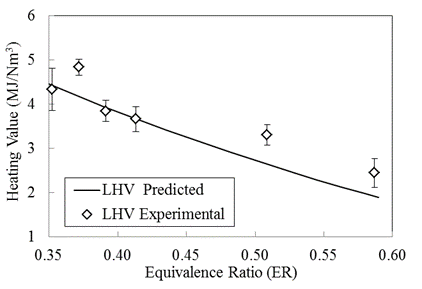Fig. 5. Comparison of predicted and experimental heating values of syngas at different equivalence ratios of gasification

Influence of Moisture Content

The influence of the initial moisture content of the OPF feedstock on the resulting syngas composition was investigated using the equilibrium model by varying the moisture content from 4% to 30% while keeping the equivalence ratio and oxidation zone temperature at 0.36 and 850 °C, respectively. Shown in Fig. 6 is the variation in the predicted composition of the major gas products in syngas with the moisture content of the fuel. As shown in Fig. 6, the concentration of CO was predicted to decrease sharply with moisture content, from the initial composition of 26% to 13%, for increases in moisture content from 4% to 30%. The composition of CO2 was predicted to increase with the increase in moisture content. The concentration of H2 was predicted to drop gradually with increases in moisture content, while the composition of CH4 was predicted to be only a trace amount at all moisture content values. The lower yield of H2 and increase in CO2 is expected as the gasification process is effective for lower moisture content feedstock, hence at higher moisture content less fuel component gases are being produced while CO2 concentration is increasing. In contrast, the relative concentration of N2 showed a rise with moisture content, probably as a result of the decrease in the overall production of other gas components for higher values of moisture content. In addition to the dry volume fractions of the permanent gases produced, the simulation results predicted an increase in H2O concentration of syngas from 9% to 20% for the moisture content range of 4% to 30%.

As expected from the predicted decreasing trends in the concentrations of the major fuel component gases CO and H2, as well as the rise in composition of CO2, the heating value of syngas decrease from 4.5 to 3 MJ/Nm3 for the increase in moisture content from 4% to 30%. This showed that operation at the lower moisture content of fuel was advantageous. A similar decreased trend in the heating value of syngas with the increase in moisture content was reported in the work of Zainal et al. (2001), where following the increase in moisture content from 0% to 40%, the syngas heating value decreased from 6 to 5.4 MJ/Nm3, 5.8 to 5.4 MJ/Nm3, and 5 to 4.5 MJ/Nm3 for the downdraft gasification of wood, paddy husk, and paper, respectively.

Shown in Table 11 is a comparison of the predicted and experimental results for the compositions of CO, CO2, H2, and N2 for the range of the initial moisture content of fuel from 10% to 28%. Experimental data points obtained from the gasification experiments for different initial moisture contents of the fuel were used for validating the model prediction results. The mean error values obtained between the predicted and experimental values for the composition of each gas component and lower heating value results are given in Table 12. From Table 11 and the mean error values given in Table 12, it could be seen that a satisfactory agreement between the predicted and experimental values was obtained for the variation of the compositions of CO, H2, and N2 with moisture content. The fraction mean error for CO2 is higher as compared to the other gas components, which could be due to lower measurement accuracy of gas analyzer.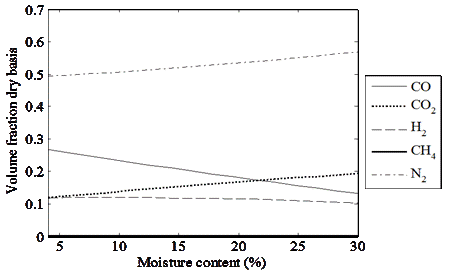Fig. 6. Moisture content of fuel versus predicted syngas composition from simulation in dry volume fraction (at ER = 0.36, Toxdn = 850 °C) for the downdraft gasification of OPF

Table 11. Comparison of Predicted and Experimental Gas Compositions for Different Fuel Moisture ContentsP* – Predicted values, E* – Experimental values

Table 12. Mean Error Fraction between Predicted and Experimental Values

 Predicted Parameters CO CO2 H2 N2 Mean Error 0.13 0.33 0.12 0.08

A comparison of the equilibrium model prediction and the experimental results for the heating value of syngas for the range of fuel moisture content between 10% and 28% is shown in Fig. 7. As shown, the predicted heating value of syngas based on the equilibrium model of gasification compared with a reasonable degree of accuracy to the experimental data. A mean error between the predicted and experimental results of only 0.09 was obtained, indicating that the model predicted the variation in the heating value of syngas with moisture content to a satisfactory level of accuracy.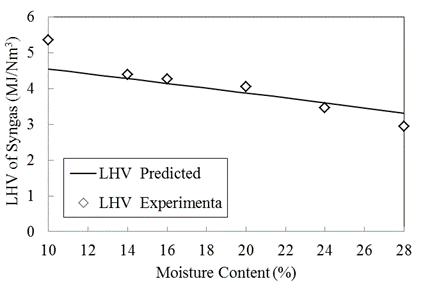Fig. 7. Comparison of predicted and experimental heating value of syngas for different fuel moisture contents

Optimization of Simulation Result

The main output parameter of interest from the biomass gasification process was the heating value of the syngas produced. The gas composition, as well as the corresponding heating value of syngas, was known to be dependent on a number of factors including operating conditions and feedstock characteristics. One common mathematical tool for optimizing a response of interest, which is dependent on a number of factors, is the response surface method (RSM) (Montgomery et al. 2009). In the present work, RSM was used to generate the mathematical relation between the response of interest, which was the heating value of syngas, and the operating conditions. Therefore, this method was used to determine the optimum conditions, namely those that would lead to syngas of the highest heating value. In the optimization, three main parameters, namely oxidation zone temperature, equivalence ratio, and moisture content, were considered in light of the objective of maximizing the heating value of the syngas produced. The data used for optimization of the three factors (temperature, moisture content, and equivalence ratio) were obtained through a number of simulation runs in which all parameters were varied together. The RSM method of optimization was performed with the help of Minitab software (Version 15, Kuantan, Malaysia), for a statistical analysis based on twenty simulation results. The selected simulation run results used in the optimization are presented in Table 13.

Table 13. Typical Simulation Results Used for Optimization using RSMAfter applying RSM on the simulation results, the empirical relationship between the response of interest (heating value) and the independent variables according to the second order model was obtained as follows,

where Y represents the heating value of the syngas, and variables x1, x2, and x3 are the values of the operating conditions, temperature (°C), moisture content (%, wet basis), and equivalence ratio, respectively, and the values of each coefficient are given in Table 14. The developed empirical relation was tested with the simulation results and was found to be comparable with a mean error of only 0.2. The optimum values identified by RSM were an oxidation zone temperature of 1000 °C, moisture content in the range of 4%, and equivalence ratio of 0.35. The corresponding optimum output of 4.82 MJ/Nm3 for the heating value was obtained with a desirability value of 0.91 (with higher value of desirability value >0.9 preferred). The optimum operating conditions identified and the resulting optimum output of the heating value were found to be in agreement with the results obtained from the experimental study.

Table 14. Values of Coefficients for Second-Order Model

 Coefficient Value0.24120.0013-0.14223.1134.5439E-070.000887979-30.2755.5952E-06-0.00330.0744

CONCLUSIONS

1. The optimization of the simulation results for maximizing the syngas heating value was performed using the Response Surface Method (RSM). This method was used to generate a mathematical relation between the response of interest (syngas heating value) and the three independent operating parameters of gasification (temperature, moisture content, and equivalence ratio). The operating condition values corresponded with the production of optimum higher heating values syngas was determined.

2. The optimum values identified by RSM were an oxidation zone temperature of 1000 °C, moisture content in the range of 4%, and equivalence ratio of 0.35.

3. The optimum operating conditions identified were comparable with the results from the experimental parametric study.

ACKNOWLEDGMENTS

The authors would like to acknowledge the financial support from the Fundamental Research Grant Scheme (FRGS) of the Malaysian Ministry of Higher Education, grant number RDU140109.

REFERENCES CITED

Atnaw, S. M., Sulaiman, S. A., and Yusup, S. (2011). “A simulation study of downdraft gasification of oil-palm fronds using ASPEN PLUS,” Journal of Applied Sciences 11(11), 1913-1920. DOI: 10.3923/jas.2011.1913.1920

Atnaw, S. M., Sulaiman, S. A., and Moni, M. N. Z. (2013a). “Experimental study on temperature profile of fixed-bed gasification of oil-palm fronds,” in: AIP Conference Proceedings Melaka, Malaysia, p. 233. DOI: 10.1063/1.4704222

Atnaw, S. M., Sulaiman, S. A., and Yusup, S. (2013b). “Syngas production from downdraft gasification of oil palm fronds,” Energy 61, 491-501. DOI: 10.1016/j.energy.2013.09.039

Atnaw, S.M., Kueh, S.C., and Sulaiman, S.A. (2014). “Study on tar generation from downdraft gasification of oil palm fronds,” The Scientific World Journal. vol. 2014, Article ID 497830, 8 pages. DOI:10.1155/2014/497830

Bates, R. B., Altantzis, C., and Ghoniem, A. F. (2016). “Modeling of biomass char gasification, combustion, and attrition kinetics in fluidized beds,” Energy & Fuels 30(1), 360-376. DOI: 10.1021/acs.energyfuels.5b02120.

Baruah, D., and Baruah, D. C. (2014). “Modeling of biomass gasification: A review,” Renewable and Sustainable Energy Reviews 39, 806-815. DOI: 10.1016/j.rser.2014.07.129

Borisov, I. I., G. G. Geîetuha, and A. A. Khalatov. (1998). “Performance and Characteristics of Wood Downdraft Gasifier with Vortex Gas Cleaning System. Biomass for Energy and Industry.” Proc. 10th European Conference and Technology Exhibition 1998.

Chang, S. H. (2014). “An overview of empty fruit bunch from oil palm as feedstock for bio-oil production,” Biomass and Bioenergy 62, 174-181. DOI: 10.1016/j.biombioe.2014.01.002

Detournay, M., Hemati, M., and Andreux, R. (2011). “Biomass steam gasification in fluidized bed of inert or catalytic particles: Comparison between experimental results and thermodynamic equilibrium predictions,” Powder Technology 208, 558-567. DOI: 10.1016/j.powtec.2010.08.059

Gautam, G. (2010). Parametric Study of a Commercial-Scale Biomass Downdraft Gasifier: Experiments and Equilibrium Modeling, Master’s thesis, Auburn University, Auburn, Alabama.

Hansen, S. B., Olsen, S. I., and Ujang, Z. (2011). “Greenhouse gas reductions through enhanced use of residues in the life cycle of Malaysian palm oil derived biodiesel,” Bioresource Technology 104, 358-366. DOI: 10.1016/j.biortech.2011.10.069

Hwang, M., Song, E.H., and Song, J.H. (2016). “One-dimensional modeling of an entrained coal gasification process using kinetic parameters,” Energies 9(2), 99. DOI: 10.3390/en9020099

Mathieu, P., and Dubuisson, R. (2002). “Performance analysis of a biomass gasifier,” Energy Conversion and Management 43(9-12), 1291-1299. DOI: 10.1016/S0196-8904(02)00015-8

Mohammed, M. A. A., Salmiaton, A., Wan Azlina, W., Mohammad Amran, M. S., Fakhru’l-Razi, A., and Taufiq-Yap, Y. H. (2010). “Hydrogen rich gas from oil palm biomass as a potential source of renewable energy in Malaysia,” Renewable and Sustainable Energy Reviews 15(2), 1258-1270. DOI: 10.1016/j.rser.2010.10.003

Montgomery, D. C., Runger, G. C., and Hubele, N. F. (2009). Engineering Statistics, Wiley and Sons, New Jersey, USA.

Muilenburg, M., Shi, Y. Y., and Ratner, A. (2011) “Computational modeling of the combustion and gasification zones in a downdraft gasifier,” ASME 2011 International Mechanical Engineering Congress and Exposition (pp. 151-158), American Society of Mechanical Engineers. DOI: 10.1115/IMECE2011-64009

Puig-Arnavat, M., Alfredo Hernandez, J., and Coronas, A. (2013) “Artificial neural network models for biomass gasification in fluidized bed gasifiers,” Biomass and Bioenergy 49 (2013), 279-289. DOI: 10.1016/j.biombioe.2012.12.012

Rajvanshi, A. K. (1986). “Biomass gasification,” Alternative Energy in Agriculture 2, 83-102.

Rodrigues, R., Muniz, A. R., and Marcilio, N. R. (2016). “Evaluation of biomass and coal co-gasification of brazilian feedstock using a chemical equilibrium model,” Brazilian Journal of Chemical Engineering 33(2), 401-414. DOI: 10.1590/0104-6632.20160332s00003479

Sulaiman, S. A., Ahmad, M. R. T., and Atnaw, S. M. (2012). “Prediction of biomass conversion process for oil palm fronds in a downdraft gasifier,” AIP Conference Proceedings, Melaka, Malaysia 1440, 981-990. DOI: 10.1063/1.4704312

Sumathi, S., Chai, S. P., and Mohamed, A. R. (2008). “Utilization of oil palm as a source of renewable energy in Malaysia,” Renewable and Sustainable Energy Reviews 12(9), 2404-2421. DOI: 10.1016/j.rser.2007.06.006.

Umar, M. S., Jennings, P., and Urmee, T. (2014). “Sustainable electricity generation from oil palm biomass wastes in Malaysia: An industry survey,” Energy 67, 496-505. DOI: 10.1016/j.energy.2014.01.067

Zainal, Z. A., Ali, R., Lean, C. H., and Seetharamu, K. N. (2001). “Prediction of performance of a downdraft gasifier using equilibrium modeling for different biomass materials,” Energy Conversion and Management 42(12), 1499-1515. DOI: 10.1016/S0196-8904(00)00078-9

Zainal, Z. A., Rifau, A., Quadir, G. A., and Seetharamu, K. N. (2002). “Experimental investigation of a downdraft biomass gasifier,” Biomass and Bioenergy 23(4), 283-289. DOI: 10.1016/S0961-9534(02)00059-4

Article submitted: October 21, 2016; Peer review completed: January 27, 2017; Revised version received and accepted: February 6, 2017; Published: February 15, 2017.

DOI: 10.15376/biores.12.2.2548-2564